# GPL Tank Level with FB

In this exercise we want to highlight the potential of the “Calculated” function available in Step 7 starting from the S7-1200 CPUs.
Consider a spherical tank for LPG gas.
We want to show on an HMI and subsequently on the SCADA the actual volume of liquefied gas inside the tank tank.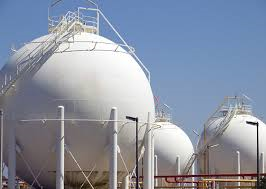The images above show typical LPG technical tanks. The stairs to climb over the tank give an idea of the actual size.
There are two main ways of proceeding, the first involves the acquisition of the load cells inserted on the pedestals of the tank, for example with the Laumas scales introduced in this text, and a second method in which the measurement of the liquid level is used. inside the tank.
In this example we will use this method.
In geometry, each of the parts in which the surface of a sphere is subdivided by a secant plane is called a spherical cap. If the secant plane passes through a diameter of the sphere, the two parts are called hemispheres. The volume between the cap and the secant plane is called the spherical segment.We need to implement these formulas using the Calculated function.
The level is shown using a bargraph on the touch. The HMI panel also shows the economic value of the stored material, calculated according to the current price per liter of LPG.
The value is entered through the appropriate field in the touch.
The system consists of a PLC model S7-1200 1212C AC / DC / rel and a KTP400 basic touch panel.
In the network they are inserted with the IPs shown in the figure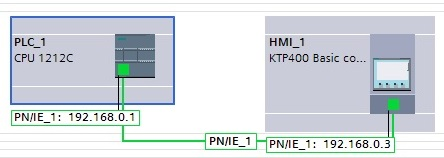In FC1 we use the calculate function.
This has the appearance of a block to which it is possible to add, by acting on the yellow star, a rather large number of topics to be used in the function we want to implement.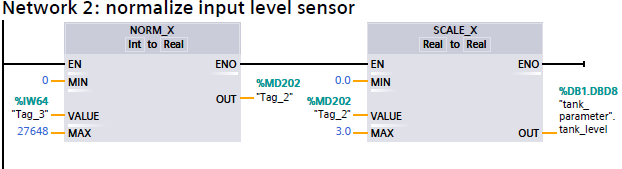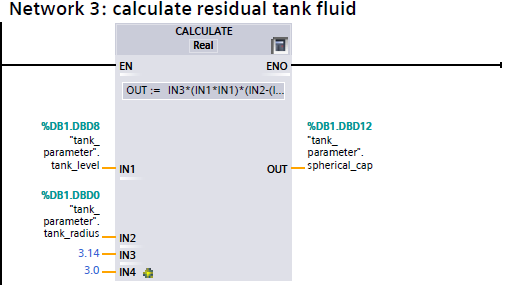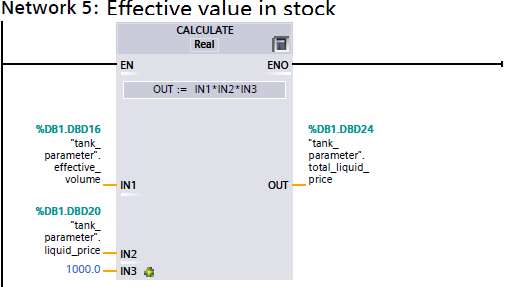By double clicking inside the calculated block, the formula setting window appears in the “OUT: =” field in which we set.The panel shows the value in cubic meters of the current content, the price in euros of the current content, and a field in which to input the current price of LPG for normal liter.
The analog channel animation shows the internal level.